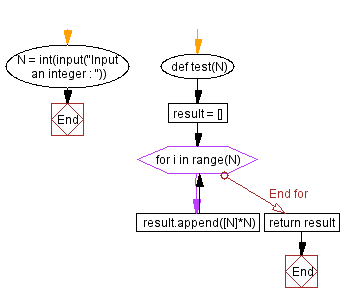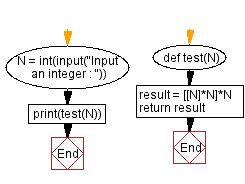﻿ Python - N x N square consisting only of the integer N

# Python Exercise: N x N square consisting only of the integer N

## Python Basic - 1: Exercise-149 with Solution

Write a Python program that takes a positive integer and creates an N x N square filled with the integer N. Display the N x N square.

Sample Data:

(2) -> [[2, 2], [2, 2]]
(5) -> [[5, 5, 5, 5, 5], [5, 5, 5, 5, 5], [5, 5, 5, 5, 5], [5, 5, 5, 5, 5], [5, 5, 5, 5, 5]]
(-6) -> []

Sample Solution-1:

Python Code:

``````def test(N):
result = []
for i in range(N):
result.append([N]*N)
return result

N = int(input("Input an integer : "))
print(test(N))``````

Sample Output:

```Input an integer :  4
[[4, 4, 4, 4], [4, 4, 4, 4], [4, 4, 4, 4], [4, 4, 4, 4]]
Input an integer :  -4
[]
```

Flowchart:Sample Solution-2:

Python Code:

``````def test(N):
result = [[N]*N]*N
return result
N = int(input("Input an integer : "))
print(test(N))
``````

Sample Output:

```Input an integer :  4
[[4, 4, 4, 4], [4, 4, 4, 4], [4, 4, 4, 4], [4, 4, 4, 4]]
```

Flowchart:Python Code Editor:

Have another way to solve this solution? Contribute your code (and comments) through Disqus.

What is the difficulty level of this exercise?

Test your Programming skills with w3resource's quiz.

﻿